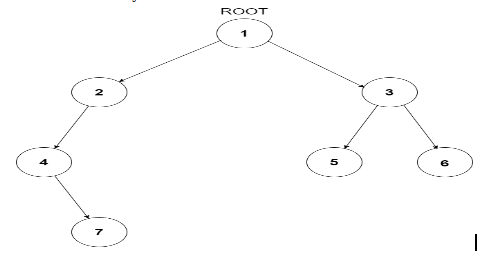# Kth ancestor of a node in binary tree

Posted: 6 Nov, 2020
Difficulty: Hard

## PROBLEM STATEMENT

#### The Kth ancestor of a node in a binary tree is the Kth node in the path going up from the given node to the root of the tree. Refer to sample test cases for further explanation.

##### Note:
``````1. The given node 'TARGET_NODE_VAL' is always present in the tree.
2. The value of each node in the tree is unique.
3. Notice that the Kth ancestor node if present will always be unique.
``````
##### Input Format:
``````The first line contains a single integer T representing the number of test cases.

The first line of each test case will contain the values of the nodes of the tree in the level order form ( -1 for NULL node) Refer to the example for further clarification.

The second line of each test case will contain two space-separated integers K and TARGET_NODE_VAL which are the value of the Kth ancestor to be found of the node and the value of the node given for which the Kth ancestor is to be computed respectively.

Example:
Consider the binary tree:
````````````The input of the tree depicted in the image above will be like:
1
2 3
4 -1 5 6
-1 7 -1 -1 -1 -1
-1 -1

Explanation :
Level 1 :
The root node of the tree is 1

Level 2 :
Left child of 1 = 2
Right child of 1 = 3

Level 3 :
Left child of 2 = 4
Right child of 2 = null (-1)
Left child of 3 = 5
Right child of 3 = 6

Level 4 :
Left child of 4 = null (-1)
Right child of 4 = 7
Left child of 5 = null (-1)
Right child of 5 = null (-1)
Left child of 6 = null (-1)
Right child of 6 = null (-1)

Level 5 :
Left child of 7 = null (-1)
Right child of 7 = null (-1)

The first not-null node (of the previous level) is treated as the parent of the first two nodes of the current level. The second not-null node (of the previous level) is treated as the parent node for the next two nodes of the current level and so on.

The input ends when all nodes at the last level are null (-1).
``````
##### Output Format:
``````For each test case, print the value of the Kth ancestor of the node in a separate line. If it doesn’t exist, then print -1.
``````
##### Note:
``````You do not need to print anything, it has already been taken care of. Just implement the given function.
``````
##### Constraints:
``````1 <= T <= 100
1 <= N <= 3000
1 <= K <= 10^9
1 <= NODE_VAL <= 10^9

Time Limit: 1 sec
``````Approach 1
• What the trivial solution suggests is that we store the parents of all the nodes till we get our target node. To do this, we can simply run a breadth-first search on the tree and traverse the tree level by level, storing the current node as the parent of its left and right child.
• Traverse the tree level order wise using a queue and keep on storing the parents of the nodes in a hashmap or any suitable data structure.
• For doing this, let us suppose that the front element of the queue is X, which is just popped from the queue,  now if X has a left child, then PAR[X→LEFT] = X. Similarly, if X has a right child then PAR[X→RIGHT] = X, where PAR[NODE] is the immediate parent of ‘NODE’.
• The 1st ancestor of node N is PAR[N]. 2nd ancestor is PAR[PAR[N]] and so on. To get the Kth parent, we can do this thing K times and keep on decrementing the value of K.
• When K becomes 0, return the parent, and if the value is -1 of the parent (i.e. we have reached the root of the tree) then return -1